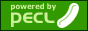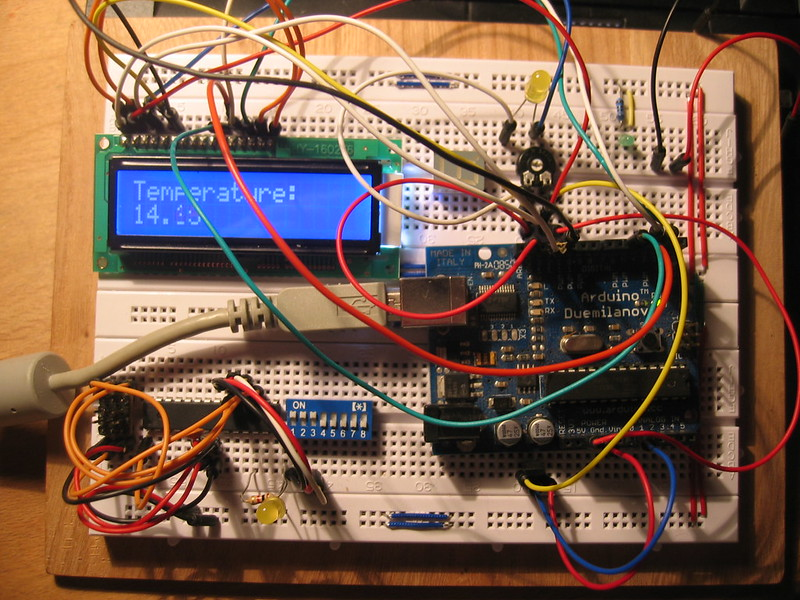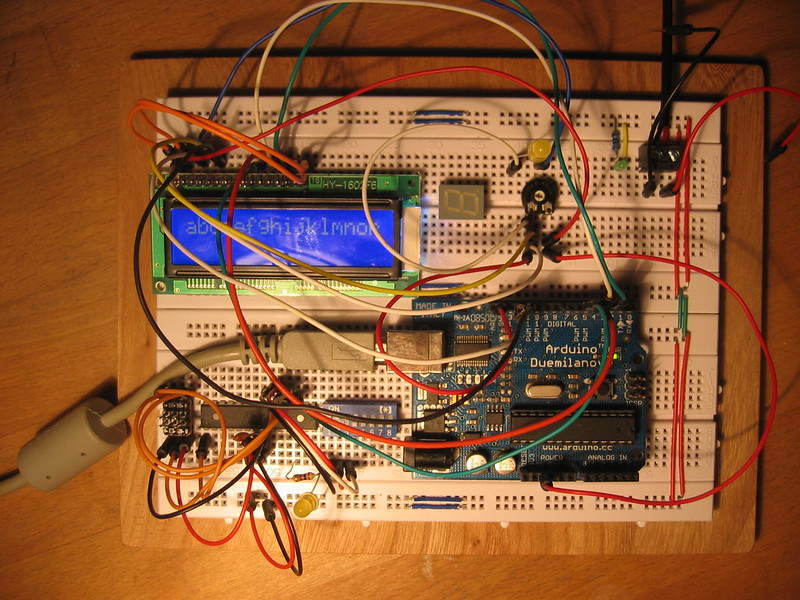## PC/SC for PHP officially released on the PECL platformI am lucky to say, that my PHP extension for using Smart Cards based on the winscard API was successful released on pecl.php.net. It is designed for the use with the wonderful project PCSC-Lite for *nix. Since PCSC-Lite is implementing the API compatible to the PC/SC standard it should be possible to compile this code on other operating systems too.

I have created this extension in 2010 but needed to clean it up and do some long term tests. It runs nicely on some machines and now you are able to do Smart Card terminal prototyping using PHP. I am using this extension to run Smart Card devices on the server side for enabling communication with Secure Application Modules (SAM) / Hardware Security Modules (HSM).

The name of the extension is "pcsc". There is only a small subset of the API implemented but for most things this is enough. I will implement the full API at any time.

To checkout the code you need to execute the following command:

`svn checkout http://svn.php.net/repository/pecl/pcsc/trunk pcsc`

Thanks to the PHP developers who helped me doing this step and for commenting my code.

## Arduino LM35 temperature sensor with LCD DisplayI added my LM35 Temperature Sensor to my Arduino again and added a display to show how hot it is... ;)

I have used the LM35 already some month ago; It is a very easy to setup device.

Here is my Code (UPADTE 2015.08.21; This is my final code.):

````// Written 2015 by Johannes Findeisen <you@hanez.org>`

`#include <LiquidCrystal.h>`

`// Initialize the LCD code. Take care of the pins I use. The first two pins`
`// are 12 and 11 in most examples around the web. I use pin 7 and 6 because of`
`// nicer wiring on my breadboard.`
`// Read this for more information:`
`// https://www.arduino.cc/en/Tutorial/LiquidCrystal`
`LiquidCrystal lcd(7, 6, 5, 4, 3, 2);`

`// Define how many samples should be collected every \$collectDelay miliseconds.`
`// After sample collection the temperature is beeing calculated and then`
`// send to the LCD.`
`int samples = 16;`

`// Collect delay between each sample.`
`int collectDelay = 2000;`

`// The pin where the status LED is connected to.`
`int ledPin = 13;`

`// The analog input pin where the LM35 is connected to.`
`int tempPin = 0;`

`// Just some variable initializations.`
`float tempC = 0;`
`float tempClast = 0;`
`float tempF = 0;`

`void setup() {`
`  pinMode(ledPin, OUTPUT);`
`  lcd.begin(16, 2);`
`  // Get data for the first time.`
`  // Read this to understand the temperature calculation:`
`  // http://www.danielandrade.net/2008/07/05/temperature-sensor-arduino/`
`  tempC = (5.0 * analogRead(tempPin) * 100.0) / 1024.0;`
`  tempClast = tempC;`
`  // Celsius to Fahrenheit conversion`
`  tempF = (tempC * 9) / 5 + 32;`
`  // Refresh the LCD output`
`  lcd_refresh();`
`}`

`void loop() {`
`  int i;`
`  tempC = 0;`
`  for(i = 0; i <= (samples - 1); i++) {`
`    tempC = tempC + ((5.0 * analogRead(tempPin) * 100.0) / 1024.0);`
`    digitalWrite(ledPin, HIGH);`
`    delay((collectDelay / 2));`
`   digitalWrite(ledPin, LOW);`
`    delay((collectDelay / 2));`
`  }`
`  // Calculate temperature`
`  tempC = tempC / (float)samples;`
`  // Celsius to Fahrenheit conversion.`
`  tempF = (tempC * 9) / 5 + 32;`
`  // Print results to the display.`
`  lcd_refresh();`
`  // Remember current temperature for next run.`
`  tempClast = tempC;`
`}`

`// Prints results to the display`
`void lcd_refresh() {`
`  lcd.setCursor(0, 0);`
`  lcd.print("Temperature");`
`  lcd.print((char)40); // ASCII code 40 = "("`
`  if(tempClast > tempC) {`
`    lcd.print((char)60); // ASCII code 60 = "<"`
`  } else if(tempClast < tempC) {`
`    lcd.print((char)62); // ASCII code 62 = ">"`
`  } else {`
`    lcd.print((char)61); // ASCII code 61 = "="`
`  }`
`  lcd.print((char)41); // ASCII code 41 = ")"`
`  lcd.print((char)58); // ASCII code 58 = ":"`
`  lcd.setCursor(0, 1);`
`  lcd.print(tempC);`
`  lcd.print((char)223); // ASCII code 223 = "°"`
`  lcd.print("C/");`
`  lcd.print(tempF);`
`  lcd.print((char)223); // ASCII code 223 = "°"`
`  lcd.print("F");`
`}````

Some other sources:

Have fun with it... ;)

## My AVR Development BoardI build a new dev board for my AVR and Arduino development. My old one really was too small... Since I am daily are working on the evaluation of all the nice and tiny IC's in my electronics box I needed this. Maybe it will become too small in some time but currently it helps me a lot.

It consists of two large breadboards and a wooden panel underneath with four rubber foots. I will work on optimizing this in the future and maybe I will write a document, describing how to build it like mine... ;)

## Pages

Page generated at Aug 30, 2023 (CET) using Jekyll on GNU/Linux @jupiter.